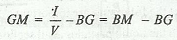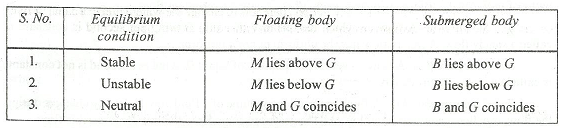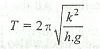# Metacentre and Metacentric Height

The Metacentre may be defined as a point about which a floating body starts oscillating, when given a small angular displacement. It is denoted by M.

The metacentric height is the distance between the centre of gravity (G) of the floating body and the metacentre (M). Mathematically, metacentric height,where

I = Moment of inertia of the sectional area of the floating body at the water surface.
V = Volume of the body submerged in water, and
BG = Distance between the centre of buoyancy (B) and the centre of gravity (G).

The conditions of equilibrium for a floating and submerged body are as follows :The time of oscillation (T) of a floating body is given bywhere

k = Radius of gyration of the floating body about its centre of gravity, and
h = Metacentric height of the floating body = GM

### You may like these posts:

#### 1 comment:

1.Its useful thank you.